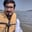Related Tags

pandas
python
communitycreator

# How to compute Euclidean distance between two series in pandasArslan Bajwa

The pandas library allows us to compute Euclidean distance between two pandas series.

## Formula

$d\left( p,q\right) = \sqrt {\sum _{i=0}^{n} \left( q_{i}-p_{i}\right)^2 }$

## Method 1: Using the formula

#importing pandas library
import pandas as pd

#initializing two pandas series
series1 = pd.Series(range(10))
series2 = pd.Series(range(100,110))

#computing the Euclidean distance using the formula
distance = sum((series1 - series2)**2)**0.5

print("Euclidean Distance: ")
print(distance)

## Method 2: Using a numpy function

#importing pandas and numpy
import pandas as pd
import numpy as np

#initializing two pandas series
series1 = pd.Series(range(10))
series2 = pd.Series(range(100,110))

#computing the Euclidan distance using a function
distance = np.linalg.norm(series1-series2)

print("Euclidean Distance: ")
print(distance)

RELATED TAGS

pandas
python
communitycreator

CONTRIBUTORArslan Bajwa
RELATED COURSES

View all Courses

Keep Exploring

Learn in-demand tech skills in half the time